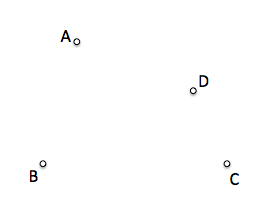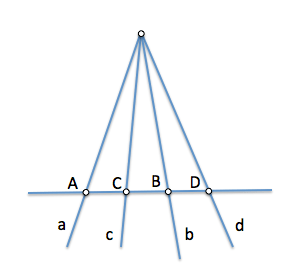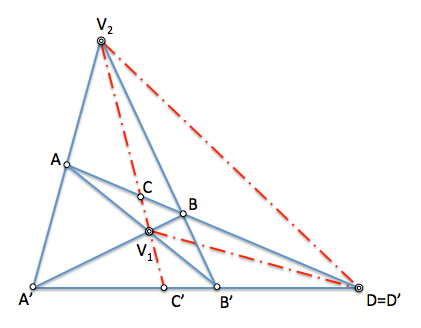One of the most used in projective geometry geometric figures is the of the “Full Cuadrivertice”, or its dual “Full ring”.

Generally, a cuadrivertice is formed by four points, so on the plane this figure has 8 degree of freedom (2 coordinates for each vertex) and they will be needed 8 restrictions to determine one concrete.This figure has 6 sides, result of joining two by two the four vertices.It contains 3 diagonal points, defined as intersections of sides that do not share a same vertex.You have 3 diagonal, each of which contains two diagonal points## Harmonics in the full Cuadrivertice relations

We will remember that given four points A, B, C and D, located on a straight line, We can define the double reason of these four points (ABCD) as the ratio of the simple reasons (ACD) and (BCD). The double reason studied it to define the quadruples of items ordered While the simple reason was formulated in the introduction to ordered triples of elements.

We similarly termed the double reason for four-straight, represented as (abcd), and we residual is why double with the points scored when sectioning these straight lines, being equal and therefore (ABCD)=(abcd)When is the value of the double reason “-1”, namely, the negative unit, We say that the elements of the Tetrad (ABCD)=(abcd)=-1 determine a harmonic Tetrad, and as a result the first two elements, points or lines, harmoniously separated both of them late each Tetrad, namely:

• It (ABCD)=-1 then “A” and “B” harmoniously separated to “C” and “D”
• It (abcd)=-1 then “to” and “b” harmoniously separated to “c” and “d”

These relationships can be found in the cuadrivertice.

If you look at the figure below, We see that (ABCD)=(A’B’C’D’) for being a same vertex V2 beam sections, but at the same time, (ABCD)=(B ’ A ’ C ’ D ’) as sections of the beam from vertex V1.It is clear from the above that (A’B’C’D’)=(B ’ A ’ C ’ D ’), but as (A’B’C’D’)= 1 /(B ’ A ’ C ’ D ’) as to the swap to’ and (B)’ Turns ratio of the triads that determine, We conclude that (ABCD)=(A’B’C’D’)=(B ’ A ’ C ’ D ’) You can only have a unitary module.

Moreover, the triplet (ACD) It should be positive for C and D on the same side with respect to, and shortlisting (BCD) It must be negative to find B from C to D.

It is clear from the last two conclusions that (ABCD)=(ACD)/(BCD) =-1 and thus the relationship is harmonious for the straight lines both points.

Two sides of a cuadrivertice separate harmonically diagonals that concur in the diagonal point that determine